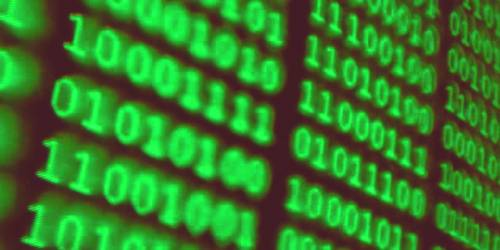# Complexity of Algorithm

Complexity of Algorithm

An algorithm is a well-defined list of steps for solving a particular problem. One major purpose of this text is to develop an efficient algorithm for the processing of our data. It is usually used for data processing, calculation, and other related computer and mathematical operations.

The analysis of an algorithm is a major task in computer science. In order to compare algorithms, we must have some criteria to measure the efficiency of algorithms. These sections discuss this important topic. The complexity of an algorithm is a measure of the amount of time and/or space required by an algorithm for an input of a given size (n).

Suppose M is an algorithm and suppose n is the size of the input data. The time and space used by the algorithm M are the two main measures for the efficiency of M. The time is measured by counting the number of key operations – in sorting and searching algorithms, For example, the number of comparisons. That is because key operations are so defined that the time for the other operations is much less than or at most proportional to the time for the key operations. Space is measured by counting the maximum of memory needed by the algorithm.

The complexity of an algorithm M is the function f(n) which gives the running time and/or storage space requirement of the algorithm in terms of the size n of the input data. Frequently the storage space required by the algorithm is simply a multiple of data size n. Accordingly, unless otherwise stated or implied the term “complexity” shall refer to the running time of the algorithm.

The following example illustrates that the function f(n) which gives the running time of an algorithm depends not only on the size n of the input data but also on the particular data.